## Subtraction in columns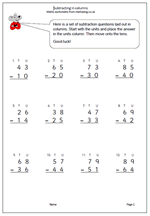It is suggested in the new Maths Programme of Study that children in Year 2 should get used to recording addition nand subtraction in columns, both to support place value and to prepare for formal written methods with larger numbers in later years.

Here we have a subtraction worksheet laid out in columns. There is no ‘borrowing’ so it is a simple matter of subtracting the units and subtracting the tens.

Subtract 2-digit numbers in columns

## New Maths Curriculum: Year 2 Addition and Subtraction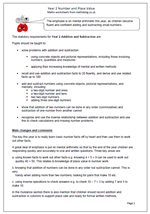Year 2 Addition and subtraction

The emphasis is on mental arithmetic this year, as children become fluent and confident adding and subtracting small numbers.

The statutory requirements for Year 2 Addition and Subtraction are:

Pupils should be taught to:

•    solve problems with addition and subtraction:

using concrete objects and pictorial representations, including those involving numbers, quantities and measures

applying their increasing knowledge of mental and written methods

•    recall and use addition and subtraction facts to 20 fluently, and derive and use related facts up to 100

•    add and subtract numbers using concrete objects, pictorial representations, and mentally, including:
a two-digit number and ones
a two-digit number and tens
two two-digit numbers

•    show that addition of two numbers can be done in any order (commutative) and subtraction of one number from another cannot

•    recognise and use the inverse relationship between addition and subtraction and use this to check calculations and missing number problems.

The key this year is to really learn basic number facts off by heart and then use them to work out other facts.
A great deal of emphasis is put on mental arithmetic so that by the end of the year children are responding quickly and accurately to oral and written questions. Three key areas are:

a. using known facts to work out other facts e.g. knowing 4 + 5 = 9 can be used to work out
quickly 40 + 50. This relates to knowledge of place value in number work.

b. knowing that addition of numbers can be done in any order but subtraction cannot. This is very
handy when adding more than two numbers; looking for pairs that make 10 etc.

c. using inverse operations to check answers e.g. to check 10 – 7 = 3 by adding 7 and 3 to make 10.

In the Guidance section there is also mention that children should record addition and subtraction in columns to support place vale and ready for formal written methods.

New Maths Programme of Study: Year 2 Addition and Subtraction

## New Maths Curriculum: Year 1 Addition and Subtraction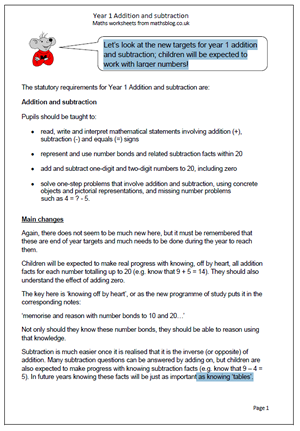Let’s look at the new targets for year 1 addition and subtraction; children will be expected to work with larger numbers!

Pupils should be taught to:

•    read, write and interpret mathematical statements involving addition (+), subtraction (-) and equals (=) signs

•    represent and use number bonds and related subtraction facts within 20

•    add and subtract one-digit and two-digit numbers to 20, including zero

•    solve one-step problems that involve addition and subtraction, using concrete objects and pictorial representations, and missing number problems
such as 4 = ? – 5.

Again, there does not seem to be much new here, but it must be remembered that these are end of year targets and much needs to be done during the year to reach them.
Children will be expected to make real progress with knowing, off by heart, all addition facts for each number totalling up to 20 (e.g. know that 9 + 5 = 14). They should also understand the effect of adding zero.
The key here is ‘knowing off by heart’, or as the new programme of study puts it in the corresponding notes:
‘memorise and reason with number bonds to 10 and 20…’
Not only should they know these number bonds, they should be able to reason using that knowledge.
Subtraction is much easier once it is realised that it is the inverse (or opposite) of addition. Many subtraction questions can be answered by adding on, but children are also expected to make progress with knowing subtraction facts (e.g. know that 9 – 4 = 5). In future years knowing these facts will be just as important as knowing ‘tables’.

New programme of Study: Year 1 Addition and Subtractionddition and subtraction

## Subtracting mentally in Year 4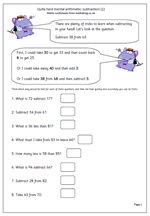Making the correct choice in deciding how to work out an answer is the key to fast mental arithmetic. Many children are unaware that there are often several ways of working out a calculation and that those who choose the best methods find maths easier and are able to answer questions more quickly and more accurately.

Let’s look at ‘Subtract 38 from 63.

This should be done, ‘in your head’ and will involve several stages, depending on the method used.

First, I could take 30 from 63 to leave 33. Then I could count back 8 from 33 which gives me 25.

Secondly, I could take 40 from 63 leaving 23 and then compensate by adding 2, which gives me 25.

Thirdly, I could take 38 from 68, leaving 30 and then compensate by subtracting 5 (because 63 is 5 less than 68).

There are several other ways very similar to these, but this does show that there is no ‘one right way, when it comes to mental arithmetic.

Quite hard mental arithmetic:_subtraction (1)

## Subtracting from whole thousands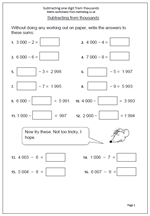Sometimes it is much easier to carry out a calculation mentally (in your head) than to use the standard written method. This page of questions illustrates this perfectly. It looks at taking a one-digit number away from a multiple of a thousand.

if this was written down on paper it would involve considerable amounts of crossing out and ‘borrowing’ from thousands to hundreds and then from hundreds to tens; a potential minefield for children. However, if they just count back from the whole thousands, even using fingers to check that they have counted back the correct number, hey presto, the answer is found in seconds.

Similarly, with a question such as 4 000 – ??? = 3993 adding on can be employed: start with 3993 and counting on until 4 000 is reached. Simple.

Subtract a single digit from thousands

## Year 1 maths worksheet: subtraction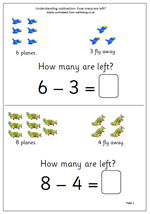It has been a while since I published anything for year 1, so here are a couple of pages on understanding subtraction. They look very straightforward but there are a number of concepts involved here:

Firstly, understanding that the phrase, ‘How many are left’ .

Secondly, relating subtraction to ‘taking away’.

Thirdly, the answer can be found by counting up from a number.

Fourthly, the answer can be found by counting back from the larger number.

Finally, that the minus sign means subtract.

Thanks to urbrainy.com for letting me use these two pages.

How many are left?

## Resource of the Week: written subtraction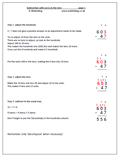This week I am looking at one of the hardest ideas to get across to children; carrying out a subtraction which requires adjusting across two columns. This only happens when there is a zero involved.

For example:

703

286 –

3 – 6 does not give a positive answer so an adjustment to the top number needs to be made. A ten can not be borrowed as there are none.

The procedure then is to borrow from the hundreds, move to the tens and then move again to the units. Often children will borrow from the hundreds, ignore the zero in the tens and move straight to the units. They cross out the 7 and make it 6 and make the 3 into 13.

This does not work as it has been 100 borrowed, not 10. Extra steps need to be taken by making the tens column 10 and then taking one ten, making this 9, and adjusting to the units.

Some children will see a quicker way: making the 70 one less (69) and adjusting to the units.

More explanation is available on the worksheet.

Standard subtraction with zeros in the tens (pg 1)

## Resource of the Week: Subtraction of money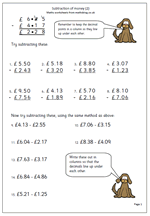The standard written method of subtraction is usually used when adding amounts of money, but it can prove quite tricky as it involves decimals. It is important to lay the question out in the standard way, even if it is shown horizontally or just as a written problem and most importantly, to keep the decimal points in a line. if using squared paper it is best to puit the decimal point on the line rather than in a square.

Watch out when there is a zero on the top line as many children go for the easy option and say ‘nought take away 3 is 3’ rather than going through the carrying process.

This is just one of our series on subtracting money.

Standard money subtraction: page 2

## Subtraction of decimals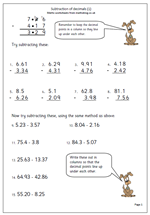Here we have another page which looks at the written method of subtracting decimals. The key here is to lay the question out in the traditional method, with the smaller number below the larger number and with the decimal points in line.

Sometimes these can be quite tricky; for example with a subtraction such as 8.5 – 6.26 it is important to write down a zero making the 8.5 into 8.50.

Also watch out for children who subtract the top number from the bottom number in an attempt to avoid the ‘decomposition’ process.

Standard subtraction of decimals (1)

## Subtracting using the standard method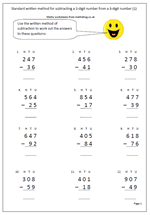Children need plenty of practice at using the standard written method of subtraction if the are going to become competent with it and calculate without making errors. This is a nice little page as it concentrates on subtracting 2-digit numbers from 3-digit numbers.

More than this the questions are graded in difficulty, according to the rows, so it also makes for an excellent assessment sheet to see just how well they are doing.

The first row is very straightforward, with no adjustments necessary.

The second row questions all require adjustment from tens to units and the third row requires adjustment from the hundreds to the tens.

The last row is the real tester as it needs adjustment from hundreds to tens to units. Watch out for the common erro, such as saying that 0 – 6 is 6.

This page is part of the collection of subtraction worksheets found in the Four Rules category.

Standard subtraction of 2-digits from 3-digits